Back to the main Index

# Third (basic) lesson with Abinit and AbiPy

Crystalline silicon.

This lesson aims at showing you how to get the following physical properties, for an insulator:

• the total energy
• the lattice parameter
• the Kohn-Sham band structure

You will learn about the use of k-points, as well as the smearing of the plane-wave kinetic energy cut-off.

This tutorial is a complement to the standard ABINIT tutorial on silicon. Here, powerful flow and visualisation procedures will be demonstrated. Still, some basic understanding of the stand-alone working of ABINIT is a prerequisite. Also, in order to fully benefit from this Abipy tutorial, other more basic Abipy tutorials should have been followed, as suggested in the abitutorials index page.

## Table of Contents¶

In :
# Use this at the beginning of your script so that your code will be compatible with python3
from __future__ import print_function, division, unicode_literals

import numpy as np

import warnings
warnings.filterwarnings("ignore")  # Ignore warnings

from abipy import abilab
abilab.enable_notebook() # This line tells AbiPy we are running inside a notebook

# This line configures matplotlib to show figures embedded in the notebook.
# Replace inline with notebook in classic notebook
%matplotlib inline

# Option available in jupyterlab. See https://github.com/matplotlib/jupyter-matplotlib
#%matplotlib widget


## Computing the total energy of silicon at fixed number of k-points¶

Our goal is to study the convergence of the total energy of silicon versus the number of k-points. So we start by defining a function that generates a Flow of SCF calculations by looping over a predefined list of ngkpt values. The crystalline structure is initialized from a CIF file while other parameters such as the cutoff energy are fixed:

In :
from lesson_base3 import build_ngkpt_flow
abilab.print_source(build_ngkpt_flow)

Out:
def build_ngkpt_flow(options):
"""
Crystalline silicon: computation of the total energy
Convergence with respect to the number of k points. Similar to tbase3_3.in

Args:
options: Command line options.

Return:
Abinit Flow object.
"""
# Definition of the different grids
ngkpt_list = [(2, 2, 2), (4, 4, 4), (6, 6, 6), (8, 8, 8)]

# These shifts will be the same for all grids
shiftk = [float(s) for s in "0.5 0.5 0.5 0.5 0.0 0.0 0.0 0.5 0.0 0.0 0.0 0.5".split()]

# Build MultiDataset object (container of ndtset inputs).
# Structure is initialized from CIF file.
multi = abilab.MultiDataset(structure=abidata.cif_file("si.cif"),
pseudos=abidata.pseudos("14si.pspnc"), ndtset=len(ngkpt_list))

# These variables are the same in each input.
multi.set_vars(ecut=8, toldfe=1e-6, diemac=12.0, iomode=3)

# Each input has its own value of ngkpt. shiftk is constant.
for i, ngkpt in enumerate(ngkpt_list):
multi[i].set_kmesh(ngkpt=ngkpt, shiftk=shiftk)

workdir = options.workdir if (options and options.workdir) else "flow_base3_ngkpt"

# Split the inputs by calling multi.datasets() and pass the list of inputs to Flow.from_inputs.
return flowtk.Flow.from_inputs(workdir, inputs=multi.split_datasets())


Let's call the function to build the flow:

In :
flow = build_ngkpt_flow(options=None)


In total, we have four ScfTasks that will be executed in the flow_base3_ngkpt directory:

In :
flow.get_graphviz()

Out:

This is the input of the first task w0_t0:

In :
flow.input

Out:
############################################################################################
# SECTION: basic
############################################################################################
ecut 8
toldfe 1e-06
ngkpt 2 2 2
kptopt 1
nshiftk 4
shiftk
0.5 0.5 0.5
0.5 0.0 0.0
0.0 0.5 0.0
0.0 0.0 0.5
############################################################################################
# SECTION: dev
############################################################################################
iomode 3
############################################################################################
# SECTION: gstate
############################################################################################
diemac 12.0
############################################################################################
# STRUCTURE
############################################################################################
natom 2
ntypat 1
typat 1 1
znucl 14
xred
0.0000000000 0.0000000000 0.0000000000
0.2500000000 0.2500000000 0.2500000000
acell 1.0 1.0 1.0
rprim
6.3285005272 0.0000000000 3.6537614829
2.1095001757 5.9665675167 3.6537614829
0.0000000000 0.0000000000 7.3075229659

and these are the divisions of the k-mesh for the four different calculations:

In :
for task in flow.iflat_tasks():
print(task.pos_str, "uses ngkpt:", task.input["ngkpt"])

w0_t0 uses ngkpt: (2, 2, 2)
w0_t1 uses ngkpt: (4, 4, 4)
w0_t2 uses ngkpt: (6, 6, 6)
w0_t3 uses ngkpt: (8, 8, 8)


but we can achieve the same goal with:

In :
flow.get_vars_dataframe("ngkpt", "ecut")

Out:
ngkpt ecut class
w0_t0 (2, 2, 2) 8 ScfTask
w0_t1 (4, 4, 4) 8 ScfTask
w0_t2 (6, 6, 6) 8 ScfTask
w0_t3 (8, 8, 8) 8 ScfTask

At this point, we could run the flow in the notebook by just calling:

flow.make_scheduler().start()



or, alternatively, execute the lesson_base3.py script to build the directory with the flow and then use:

abirun.py flow_base3_ngkpt scheduler



inside the terminal.

## Analysis of the results¶

We could use the API provided by the flow to extract the total energies from the GSR files. Something like:

nkpt_list, ene_list = [], []
for task in flow.iflat_tasks():
with task.open_gsr() as gsr:
nkpt_list.append(gsr.nkpt)
ene_list.append(gsr.energy)

# Assuming values are already sorted wrt nkpt
import matplotlib.pyplot as plt
plt.plot(nkpt_list, ene_list, marker="o");


but it is much easier to create a GsrRobot that will do the work for us:

In :
robot_enekpt = abilab.GsrRobot.from_dir("flow_base3_ngkpt")
robot_enekpt

Out:
1. w0/t0/outdata/out_GSR.nc
2. w0/t1/outdata/out_GSR.nc
3. w0/t2/outdata/out_GSR.nc
4. w0/t3/outdata/out_GSR.nc

In the next lines, we are going to generate a pandas Dataframe with the most important results so that we can show how to use the pandas API to analyze the data:

In :
ene_table = robot_enekpt.get_dataframe()
ene_table.keys()

Out:
Index(['formula', 'natom', 'alpha', 'beta', 'gamma', 'a', 'b', 'c', 'volume',
'abispg_num', 'spglib_symb', 'spglib_num', 'spglib_lattice_type',
'energy', 'pressure', 'max_force', 'ecut', 'pawecutdg', 'tsmear',
'nkpt', 'nsppol', 'nspinor', 'nspden'],
dtype='object')

The dataframe contains several columns but we are mainly interested in the number of k-points nkpt and in the energy (given in eV). Let's massage a bit the data to facilitate the post-processing:

In :
# We are gonna plot f(nkpt) so let's sort the rows first.
ene_table.sort_values(by="nkpt", inplace=True)

# Add a column with energies in Ha and another column with the difference wrt to the last point.
ene_table["energy_Ha"] = ene_table["energy"] * abilab.units.eV_to_Ha
ene_table["ediff_Ha"] = ene_table["energy_Ha"] - ene_table["energy_Ha"][-1]


before printing a subset of the columns with the syntax:

In :
ene_table[["nkpt", "energy", "energy_Ha", "ediff_Ha"]]

Out:
nkpt energy energy_Ha ediff_Ha
w0/t0/outdata/out_GSR.nc 2 -241.251546 -8.865831 0.006242
w0/t1/outdata/out_GSR.nc 10 -241.417959 -8.871946 0.000126
w0/t2/outdata/out_GSR.nc 28 -241.421158 -8.872064 0.000009
w0/t3/outdata/out_GSR.nc 60 -241.421391 -8.872073 0.000000

If you do not like tables and prefer figures, use:

In :
ene_table.plot(x="nkpt", y=["energy_Ha", "ediff_Ha", "pressure"], style="-o", subplots=True);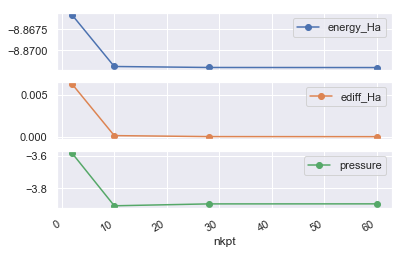The difference between dataset 3 and dataset 4 is rather small. Even the dataset 2 gives an accuracy of about 0.0001 Ha. So, our converged value for the total energy (at fixed acell and ecut) is -8.8726 Ha.

Now that we have learned a bit how to use pandas Dataframes, we can finally reveal that the AbiPy robots already provide methods to perform this kind of convergence studies so that we do not need to manipulate pandas dataframes explicitly. For example, we can perform the same analysis with a single line:

In :
robot_enekpt.plot_gsr_convergence(sortby="nkpt");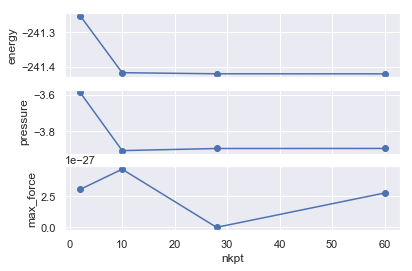We can also pass a function that will be called by the robot to compute the values along the x-axis and sort the results. The docstring of the function is used as label of the x-axis:

In :
def inv_nkpt(abifile):
r"""$\dfrac{1}{nkpt}$"""
return 1 / abifile.nkpt

robot_enekpt.plot_gsr_convergence(sortby=inv_nkpt);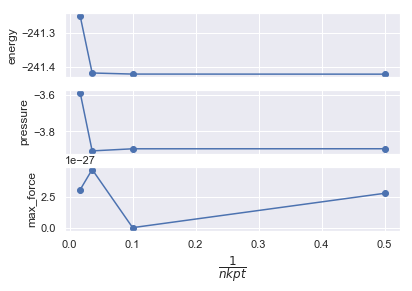In :
#robot_enekpt.plot_lattice_convergence(sortby="nkpt");


## Determination of the lattice parameters¶

At this point, the original Abinit tutorial proceeds with a convergence study for the optimized lattice parameters as function of the k-point sampling. In AbiPy, we only need to build different relaxation tasks with a slightly different input in which only ngkpt is changed.

In :
from lesson_base3 import build_relax_flow
abilab.print_source(build_relax_flow)

Out:
def build_relax_flow(options):
"""
Crystalline silicon: computation of the optimal lattice parameter.
Convergence with respect to the number of k points. Similar to tbase3_4.in
"""
# Structural relaxation for different k-point samplings.
ngkpt_list = [(2, 2, 2), (4, 4, 4)]

shiftk = [float(s) for s in "0.5 0.5 0.5 0.5 0.0 0.0 0.0 0.5 0.0 0.0 0.0 0.5".split()]

multi = abilab.MultiDataset(structure=abidata.cif_file("si.cif"),
pseudos=abidata.pseudos("14si.pspnc"), ndtset=len(ngkpt_list))

# Global variables
multi.set_vars(
ecut=8,
tolvrs=1e-9,
optcell=1,
ionmov=3,
ntime=10,
dilatmx=1.05,
ecutsm=0.5,
diemac=12,
iomode=3,
)

for i, ngkpt in enumerate(ngkpt_list):
multi[i].set_kmesh(ngkpt=ngkpt, shiftk=shiftk)

workdir = options.workdir if (options and options.workdir) else "flow_base3_relax"

return flowtk.Flow.from_inputs(workdir, inputs=multi.split_datasets(), task_class=flowtk.RelaxTask)

In :
relax_flow = build_relax_flow(options=None)
relax_flow.get_graphviz()

Out:
If you want to run the flow from the shell, open lesson_base3.py and change the main function so that it calls build_relax_flow instead of build_ngkpt_flow.

This is our first structural relaxation with AbiPy and this gives us the opportunity to introduce the HIST.nc file. This file stores the history of the relaxation (energies, forces, stresses, lattice parameters and atomic positions at the different relaxation steps).

As usual, we use abiopen to generate an AbiPy object:

In :
hist = abilab.abiopen("flow_base3_relax/w0/t1/outdata/out_HIST.nc")
print(hist)

================================= File Info =================================
Name: out_HIST.nc
Directory: /Users/gmatteo/git_repos/abitutorials/abitutorials/base3/flow_base3_relax/w0/t1/outdata
Size: 4.24 kb
Access Time: Mon Aug 13 16:49:10 2018
Modification Time: Wed Oct 11 00:28:35 2017
Change Time: Wed Oct 11 00:28:35 2017

============================= Initial Structure =============================
Full Formula (Si2)
Reduced Formula: Si
abc   :   3.866975   3.866975   3.866975
angles:  60.000000  60.000000  60.000000
Sites (2)
#  SP       a     b     c  cartesian_forces
---  ----  ----  ----  ----  -----------------------------------------------------------
0  Si    0     0     0     [ 4.10231606e-27 -5.80155101e-27 -3.31586260e-27] eV ang^-1
1  Si    0.25  0.25  0.25  [-4.10231606e-27  5.80155101e-27  3.31586260e-27] eV ang^-1

Number of relaxation steps performed: 4
============================== Final structure ==============================
Full Formula (Si2)
Reduced Formula: Si
abc   :   3.822962   3.822962   3.822962
angles:  60.000000  60.000000  60.000000
Sites (2)
#  SP       a      b     c  cartesian_forces
---  ----  ----  -----  ----  -----------------------------------------------------------
0  Si    0     -0     0     [5.53272686e-28 6.25956589e-27 9.58296402e-28] eV ang^-1
1  Si    0.25   0.25  0.25  [-5.53272686e-28 -6.25956589e-27 -9.58296402e-28] eV ang^-1

Volume change in percentage: -3.38%
Percentage lattice parameter changes:
a: -1.14%, b: -1.14%, c: -1%

Stress tensor (Cartesian coordinates in GPa):
[[8.75674654e-03 5.30998779e-11 7.50894518e-11]
[5.30998779e-11 8.75674654e-03 5.30995448e-11]
[7.50894518e-11 5.30995448e-11 8.75674652e-03]]
Pressure: -0.009 [GPa]


To plot the evolution of the most important physical quantities, use:

In :
hist.plot();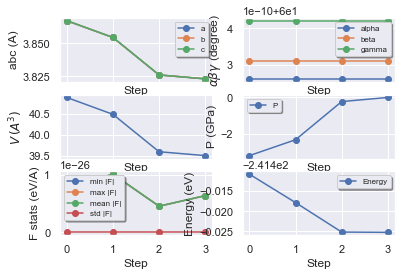In :
hist_robot = abilab.HistRobot.from_dir("flow_base3_relax")

hist_table = hist_robot.get_dataframe()


There are several entries in the DataFrame:

In :
hist_table.keys()

Out:
Index(['formula', 'natom', 'alpha', 'beta', 'gamma', 'a', 'b', 'c', 'volume',
'abispg_num', 'spglib_symb', 'spglib_num', 'spglib_lattice_type',
'num_steps', 'final_energy', 'final_pressure', 'final_fmin',
'final_fmax', 'final_fmean', 'final_fstd', 'final_drift',
'initial_fmin', 'initial_fmax', 'initial_fmean', 'initial_fstd',
'initial_drift'],
dtype='object')

Let's select some of them with:

In :
hist_table[["alpha", "a", "final_energy", "final_pressure", "num_steps"]]

Out:
alpha a final_energy final_pressure num_steps
w0/t0/outdata/out_HIST.nc 60.0 3.829282 -241.255628 -0.013259 4
w0/t1/outdata/out_HIST.nc 60.0 3.822962 -241.425215 -0.008757 4

and print the evolution of important physical properties extracted from the two files:

In :
hist_robot.gridplot(what_list=["energy", "abc", "pressure", "forces"]);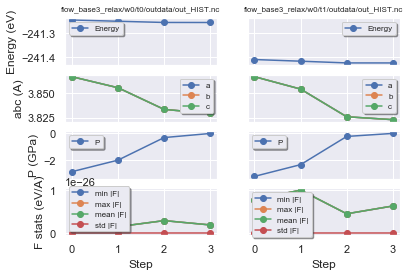We can also compare the two structural relaxations with:

In :
hist_robot.combiplot();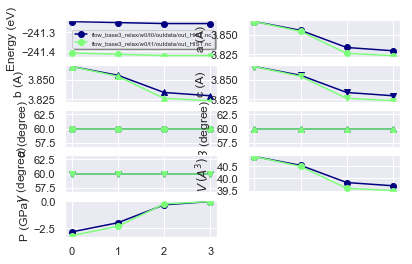Unfortunately the HIST.nc file does not have enough metadata. In particular we would like to have information about the k-point sampling so that we can analyze the convergence of the optimized lattice parameters wrt nkpt. Fortunately the GSR.nc has all the information we need and it is just a matter of replacing the HistRobot with a GsrRobot:

In :
with abilab.GsrRobot.from_dir("flow_base3_relax") as relkpt_robot:
relax_table = relkpt_robot.get_dataframe().sort_values(by="nkpt")
dfs = relkpt_robot.get_structure_dataframes()

In :
relax_table[["energy", "a", "pressure", "max_force", "pressure"]]

Out:
energy a pressure max_force pressure
w0/t0/outdata/out_GSR.nc -241.255628 3.829282 -0.013259 1.913429e-27 -0.013259
w0/t1/outdata/out_GSR.nc -241.425215 3.822962 -0.008757 6.356619e-27 -0.008757

Plotting the energy, the lattice parameter a in Bohr and the pressure in GPa vs nkpt is really a piece of cake!

In :
relax_table.plot(x="nkpt", y=["energy", "a", "pressure"], subplots=True);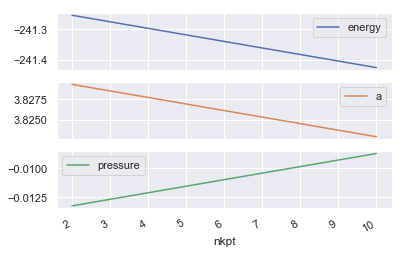Alternatively, one can use the GsrRobot API:

In :
relkpt_robot.plot_gsr_convergence(sortby="nkpt");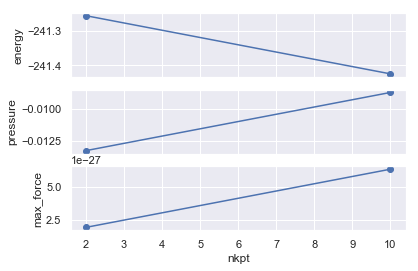In :
relkpt_robot.plot_lattice_convergence(what_list=["a"], sortby="nkpt");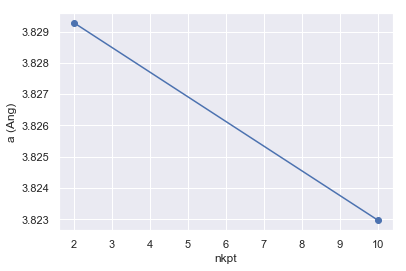We fix the parameters acell to the theoretical value of 3*10.216, and we fix also the grid of k points (the 4x4x4 FCC grid, equivalent to a 8x8x8 Monkhorst-pack grid). We will ask for 8 bands (4 valence and 4 conduction).

## Computing the band structure¶

A band structure can be computed by solving the Kohn-Sham equation for many different k points, along different lines of the Brillouin zone. The potential that enters the Kohn-Sham equation must be derived from a previous self-consistent calculation, and will not vary during the scan of different k-point lines.

This is our first Flow with dependencies in the sense that the band structure calculation must be connected to a previous SCF run. Fortunately AbiPy provides a factory function to generate this kind of workflow. We only need to focus on the definition of the two inputs:

In :
from lesson_base3 import build_ebands_flow
abilab.print_source(build_ebands_flow)

Out:
def build_ebands_flow(options):
"""
Band structure calculation.
First, a SCF density computation, then a non-SCF band structure calculation.
Similar to tbase3_5.in
"""
multi = abilab.MultiDataset(structure=abidata.cif_file("si.cif"),
pseudos=abidata.pseudos("14si.pspnc"), ndtset=2)
# Global variables
shiftk = [float(s) for s in "0.5 0.5 0.5 0.5 0.0 0.0 0.0 0.5 0.0 0.0 0.0 0.5".split()]
multi.set_vars(ecut=8, diemac=12, iomode=3)

# Dataset 1
multi.set_vars(tolvrs=1e-9)
multi.set_kmesh(ngkpt=[4, 4, 4], shiftk=shiftk)

# Dataset 2
multi.set_vars(tolwfr=1e-15)
multi.set_kpath(ndivsm=5)

scf_input, nscf_input = multi.split_datasets()

workdir = options.workdir if (options and options.workdir) else "flow_base3_ebands"

return flowtk.bandstructure_flow(workdir, scf_input=scf_input, nscf_input=nscf_input)


The Flow consists of a single Work with two Tasks (ScfTask with a k-mesh and a NscfTask performed on the k-path).

In :
ebands_flow = build_ebands_flow(options=None)
ebands_flow.get_graphviz()

Out:
If you want to run the flow from the shell, open lesson_base3.py and change the main function so that it calls build_ebands_flow.

Let's extract the band structure from the GSR.nc file produced by the NscfTask:

In :
with abilab.abiopen("flow_base3_ebands/w0/t1/outdata/out_GSR.nc") as gsr:
ebands_kpath = gsr.ebands


and plot it with:

In :
ebands_kpath.plot();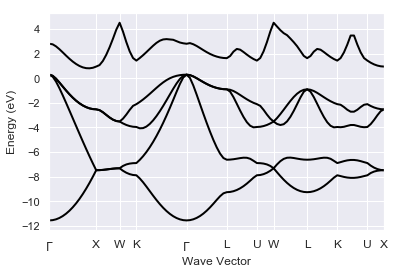Visual inspection reveals that the width of the valence band is ~11.8 eV, the lowest unoccupied state at X is ~0.5 eV higher than the top of the valence band at $\Gamma$. Bulk silicon is described as an indirect band gap material (this is correct), with a band-gap of about 0.5 eV (this is quantitatively quite wrong: the experimental value is 1.17 eV at 25 degree Celsius, the famous DFT band-gap problem). The minimum of the conduction band is even slightly displaced with respect to X.

Unfortunately, it seems that AbiPy does not agree with us:

In :
print(ebands_kpath)

================================= Structure =================================
Full Formula (Si2)
Reduced Formula: Si
abc   :   3.866975   3.866975   3.866975
angles:  60.000000  60.000000  60.000000
Sites (2)
#  SP       a     b     c
---  ----  ----  ----  ----
0  Si    0     0     0
1  Si    0.25  0.25  0.25

Abinit Spacegroup: spgid: 227, num_spatial_symmetries: 48, has_timerev: True, symmorphic: True

Number of electrons: 8.0, Fermi level: 5.280 (eV)
nsppol: 1, nkpt: 101, mband: 5, nspinor: 1, nspden: 1
smearing scheme: none, tsmear_eV: 0.272, occopt: 1
Direct gap:
Energy: 0.309 (eV)
Initial state: spin=0, kpt=[+0.050, +0.050, +0.100], weight: 0.000, band=2, eig=5.218, occ=2.000
Final state:   spin=0, kpt=[+0.050, +0.050, +0.100], weight: 0.000, band=3, eig=5.527, occ=2.000
Fundamental gap:
Energy: 0.021 (eV)
Initial state: spin=0, kpt=[+0.125, +0.125, +0.125], weight: 0.000, band=3, eig=5.280, occ=2.000
Final state:   spin=0, kpt=[+0.042, +0.042, +0.042], weight: 0.000, band=1, eig=5.301, occ=2.000
Bandwidth: 11.564 (eV)
Valence maximum located at:
spin=0, kpt=[+0.125, +0.125, +0.125], weight: 0.000, band=3, eig=5.280, occ=2.000
Conduction minimum located at:
spin=0, kpt=[+0.042, +0.042, +0.042], weight: 0.000, band=1, eig=5.301, occ=2.000



The reason is that ther Fermi energy in ebands_kpath is not completely consistent with the band structure. The Fermi energy, indeed, has been taken from the previous GS-SCF calculation performed on a shifted k-mesh, the $\Gamma$ point was not included and therefore the Fermi energy is underestimated.

To fix this problem we have to change manually the Fermi energy and set it to the maximum of the valence bands:

In :
ebands_kpath.set_fermie_to_vbm()
print(ebands_kpath)

================================= Structure =================================
Full Formula (Si2)
Reduced Formula: Si
abc   :   3.866975   3.866975   3.866975
angles:  60.000000  60.000000  60.000000
Sites (2)
#  SP       a     b     c
---  ----  ----  ----  ----
0  Si    0     0     0
1  Si    0.25  0.25  0.25

Abinit Spacegroup: spgid: 227, num_spatial_symmetries: 48, has_timerev: True, symmorphic: True

Number of electrons: 8.0, Fermi level: 5.567 (eV)
nsppol: 1, nkpt: 101, mband: 5, nspinor: 1, nspden: 1
smearing scheme: none, tsmear_eV: 0.272, occopt: 1
Direct gap:
Energy: 2.512 (eV)
Initial state: spin=0, kpt=[+0.000, +0.000, +0.000], name: $\Gamma$, weight: 0.000, band=3, eig=5.567, occ=2.000
Final state:   spin=0, kpt=[+0.000, +0.000, +0.000], name: $\Gamma$, weight: 0.000, band=4, eig=8.080, occ=0.000
Fundamental gap:
Energy: 0.517 (eV)
Initial state: spin=0, kpt=[+0.000, +0.000, +0.000], name: $\Gamma$, weight: 0.000, band=3, eig=5.567, occ=2.000
Final state:   spin=0, kpt=[+0.429, +0.000, +0.429], weight: 0.000, band=4, eig=6.084, occ=0.000
Bandwidth: 11.852 (eV)
Valence maximum located at:
spin=0, kpt=[+0.000, +0.000, +0.000], name: $\Gamma$, weight: 0.000, band=3, eig=5.567, occ=2.000
Conduction minimum located at:
spin=0, kpt=[+0.429, +0.000, +0.429], weight: 0.000, band=4, eig=6.084, occ=0.000



Now the AbiPy results are consistent with our initial analysis:

In :
ebands_kpath.plot(with_gaps=True);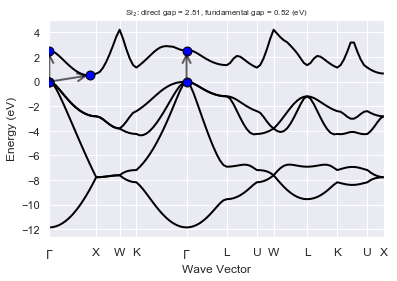In :
# We can also plot the k-path in the Brillouin zone with:
#ebands_kpath.kpoints.plot();


The GSR file produced by the first task contains energies on a homogeneous k-mesh. We can therefore compute the DOS by invoking the get_edos method:

In :
with abilab.abiopen("flow_base3_ebands/w0/t0/outdata/out_GSR.nc") as gsr:
ebands_kmesh = gsr.ebands

edos = ebands_kmesh.get_edos()


and plot the DOS with:

In :
edos.plot();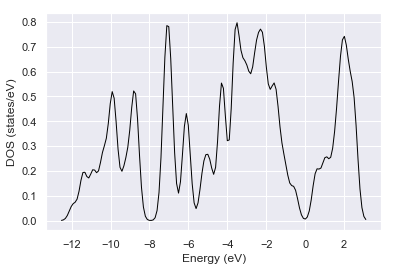where the zero of the energy axis is set to the Fermi level $\epsilon_F$ obtained by solving:

$$\int_{-\infty}^{\epsilon_F} g(\epsilon)\,d\epsilon = N$$

for $\epsilon_F$ with $N$ the number of electrons per unit cell. Note that the DOS is highly sensitive to the sampling of the IBZ and to the value of the broadening, especially in metallic systems.

In :
edos.plot_dos_idos();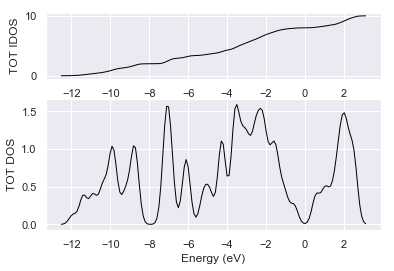Want to make a nice picture of the band dispersion with a second panel for the DOS?

In :
ebands_kpath.plot_with_edos(edos);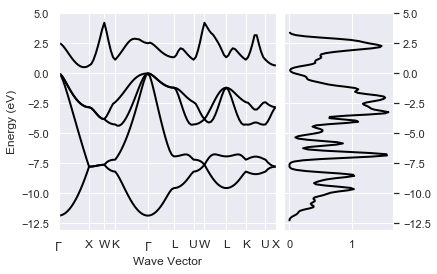It is important to stress that each panel in the above figure is aligned with respect to its own Fermi energy and these values are not necessarly equal:

In :
print(ebands_kpath.fermie, edos.fermie)

5.567493548211215 eV 5.838293265593386


We can always plot the bands and the DOS without setting their Fermi energy to zero by using:

In :
ebands_kpath.plot_with_edos(edos, e0=0);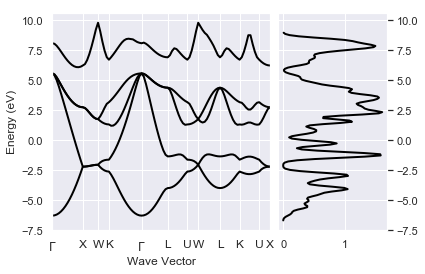This figure shows that the bands and the DOS are not perfectly aligned. More specifically we would expect the DOS to be zero at the bottom/top of the conduction. This problems is essentially due to the use of a relatively large gaussian broadening. One should therefore compute the DOS with a much denser IBZ mesh and a much smaller broadening to solve this alignment issue

Back to the main Index

In [ ]: